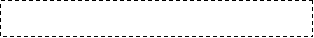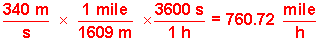Name:                                                     Sec.# ( 8) ---Quiz ( 1)-Ch(1)                                  S.N:

ID :                                                       Phys 101 ( Term 041)-( F. Enaya)Show your steps clearly for full credit.!!

Q.1: The final velocity of a drop of water falling through the atmosphere is given by: v = (2/9)(r2 g ρ/ n) where v is the velocity of the drop in m/s; r is the radius in m; g is the acceleration due the gravity in m/s2; and ρ is the density of the water in kg/m3. What must be the unit of n, the coefficient of the velocity of the air which the drop falls?

In units :

n =  ( r2 g r )/ v  =  (m2 .  m . kg/m3)/(m/s) = (kg/s . m )

Q.2:  Speed of the sound is 340 m/s. Express this in mile per hour. ( 1 mile = 1609 m)